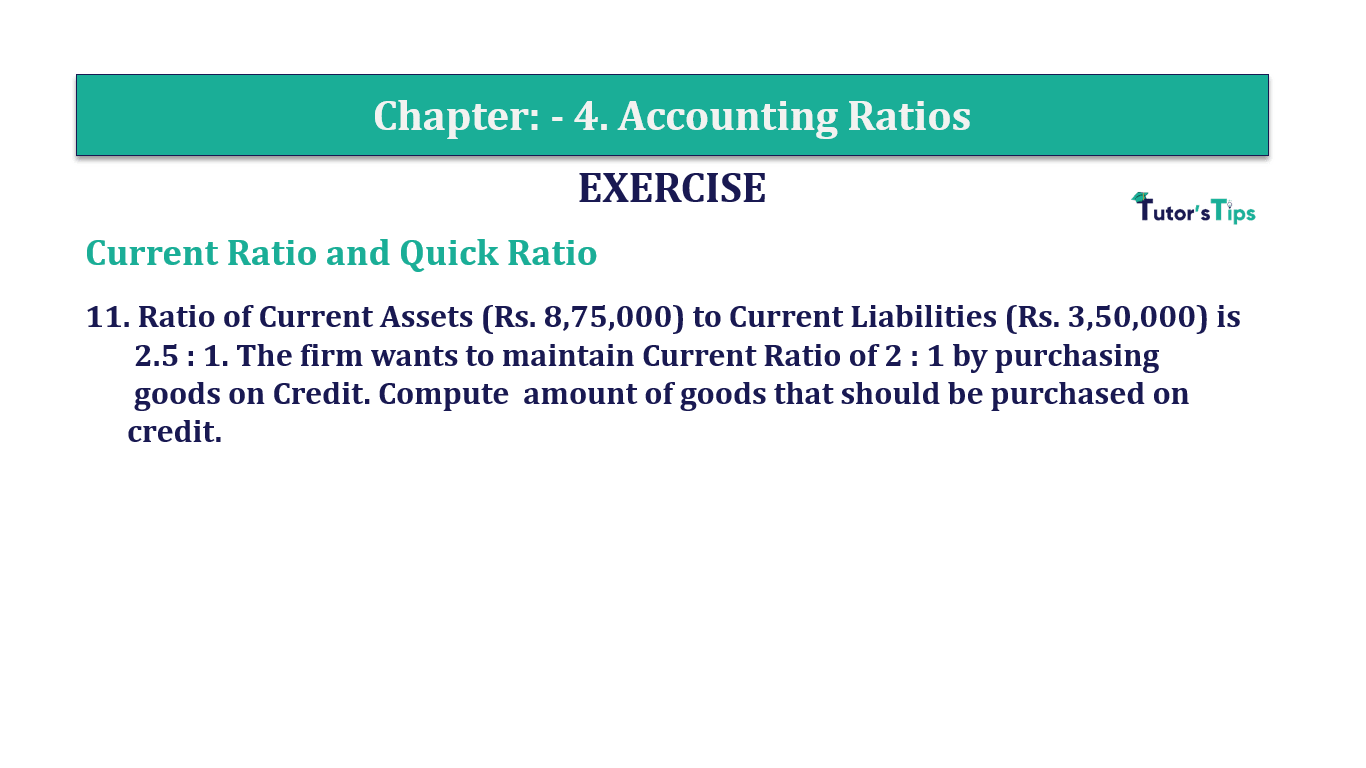# Question 11 Chapter 4 of +2-B – T.S. Grewal 12 ClassQuestion No. 11 - Chapter No.4 - T.S. Grewal +2 Book Part B

Question 11 Chapter 4 of +2-B

Current Ratio and Quick Ratio

11. Ratio of Current Assets (Rs. 8,75,000) to Current Liabilities (Rs. 3,50,000) is 2.5 : 1. The firm wants to maintain a Current Ratio of 2: 1 by purchasing
goods on Credit. Compute the number of goods that should be purchased on
credit.

### The solution of Question 11 Chapter 4 of +2-B: –

 Current Liabilities =Rs. 3,50,000
 Current Assets =Rs. 8,75,000
 Current Ratio =2.5: 1

The firm is interested to maintain the Current Ratio 2: 1 by Purchasing goods on credit.
∴ Let the number of goods purchased on credit be = x

 Current Liabilities =Rs. 3,50,000 + x
 Current Assets =Rs. 8,75,000 + x

 Current Ratio = Current Assets = Rs. 8,75,000 = 2 Current Liabilities Rs. 3,50,000 1
 Rs. 8,75,000 + x Rs. 7,00,000 + 2x

Rs. 8,75,000 – Rs. 7,00,000  2x-x

Rs. 1,75,000  x

Therefore, goods worth Rs.1,75,000 must be purchased on credit to maintain the current ratio at 2:1

Balance Sheet: Meaning, Format & Examples

Comment if you have any question.

Also, Check out the solved question of previous Chapters: –